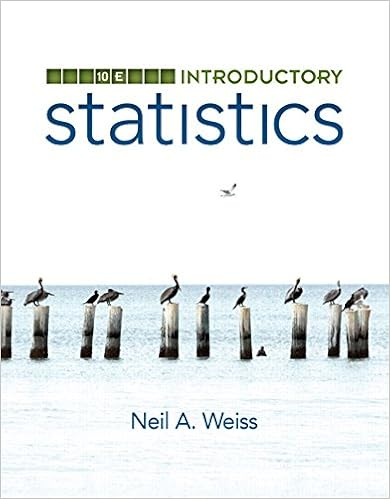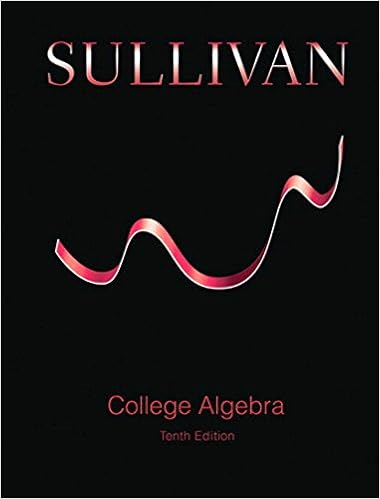# Solutions10 - Math 601 Solutions to Homework 10 1. Consider...

• Homework Help
• 7

This preview shows page 1 - 3 out of 7 pages.

The preview shows page 1 - 3 out of 7 pages.
Math 601 Solutions to Homework 101. Consider the vector fieldF(x, y) = (2x+ 2y)i+ (2x-y)j.(a) Find the parametric equations for the flow line ofFbeginning atthe point (0,5).(b) Find div(F).(c) Find rot(F).Answer:(a) We need to solve the system of differential equations:dxdt=2x+ 2ydydt=2x-yThis is a system of linear differential equations, which we can writeas:ddtxy=222-1‚ •xyTo solve this system, we need to compute the eigenvalues andeigenvectors of the matrix.We can quickly find the eigenvalues if we remember that the prod-uct of the eigenvalues must equal the determinant of the matrix,and the sum of the eigenvalues must equal the trace of the matrix.So,λ1λ2=-6 andλ1+λ2= 1. By inspection we see that theeigenvalues areλ1= 3 andλ2=-2. (This method only works for2×2 matrices.)Now, we find the eigenvectors associated with each eigenvalue.Forλ1= 3, we have:nullspaceλ1-2-2-2λ1+ 1=nullspace1-2-24=nullspace1-200=Span21‚¶Thus, the vector21is an eigenvector associated with the eigen-valueλ1= 3.
##### We have textbook solutions for you!The document you are viewing contains questions related to this textbook.
Chapter 9 / Exercise 11-14
Precalculus: Mathematics for Calculus
Redlin/StewartExpert VerifiedBrowse all Textbook Solutions
Forλ2=-2, we have:nullspaceλ2-2-2-2λ2+ 1=nullspace-4-2-2-1=nullspace2100=Span1-2‚¶Thus, the vector1-2is an eigenvector associated with theeigenvalueλ2=-2.Thus, the general solution to the system of linear differential equa-tions is:xy=c1e3t21+c2e-2t1-2We would like to find the solution which begins at the point (0,5),so we wantx= 0, y= 5 whent= 0. We can plug those num-bers into the above equation and solve forc1andc

Course Hero member to access this document

Course Hero member to access this document

End of preview. Want to read all 7 pages?

Course Hero member to access this document

Term
Spring
Professor
alndy
Tags
Eigenvalue eigenvector and eigenspace, dr d, Parametric equation
##### We have textbook solutions for you!
The document you are viewing contains questions related to this textbook.The document you are viewing contains questions related to this textbook.
Chapter 9 / Exercise 11–14
Precalculus: Mathematics for Calculus
Redlin/StewartExpert Verified
•••